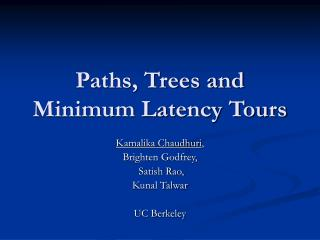DownloadDownload PresentationPaths, Trees and Minimum Latency Tours

# Paths, Trees and Minimum Latency Tours

Télécharger la présentation## Paths, Trees and Minimum Latency Tours

- - - - - - - - - - - - - - - - - - - - - - - - - - - E N D - - - - - - - - - - - - - - - - - - - - - - - - - - -
##### Presentation Transcript

1. Paths, Trees and Minimum Latency Tours Kamalika Chaudhuri, Brighten Godfrey, Satish Rao, Kunal Talwar UC Berkeley

2. The Problem • Given: • V: Set of points • d : Distance function on pairs of points • s : Starting point Find a tour of all points, starting at s, which minimizes the total latency • Also called the Traveling Repairman problem

3. Some Results • [SG74] NP-Hard on general graphs • [Sitters02] NP Hard on weighted trees • [BCCPRS94] MAX-SNP Hard on general graphs • [BCCPRS94] Constant factor algorithm for metric spaces • [GK96] Approximation ratio : 7.18 + ε • Our approximation ratio : 3.59

4. An Algorithm [BCCPRS94] • For j=1,2,3,.. • Find a tree Tj of cost at most 2j which has the most vertices (*) • Double Tj and shortcut to get tour Pj • Concatenate tours P1,P2,…

5. Analysis • Suppose 2j·latencyOPT(i)· 2j+1 • Tj+1 has at least i vertices • Latency of the ith vertex in our tour is at most • 2 £ 2j+1 + 2 k 2k • · 8 latencyOPT(i) • Problem: • Assumed that we can find exact solution to k-MST (the minimum spanning tree with k vertices) •  approximate k-MSTs : approximation factor 8 

6. [BCCPRS94], [GK96] Lower bound: k-MST Tours from k-MSTs Tours of geometrically increasing lengths 3.59  ¼ 7.18 approximation Our Algorithm Lower bound: k-stroll Tours fromgood k-trees Tours of geometrically increasing lengths 3.59 approximation Trees vs. Paths

7. Our Algorithm Lower bound: k-stroll Tours fromgood k-trees Tours of geometrically increasing lengths 3.59 Approximation Trees vs. Paths This talk: • k-(stroll, tree) • Finding good k-trees

8. Paths vs. Trees • Our contribution: • Use k-stroll as a lower bound instead of k-MST k-stroll : Given s, the minimum cost path from s with k vertices But k-stroll does not seem any easier than k-MST !

9. Good k-trees Good k-tree : • k vertices • Tree cost · optimal k-stroll Find a good k-tree by a modification of the k-MST algorithm [Garg96,AK00]

10. Finding good k-trees • [Garg96, AK00] use a variant of the primal-dual algorithm of [GW92] • Allot a budget  to each vertex • Different  s produce trees of different size k

11. Finding good trees • Our algorithm: • Fix endpoint t • Budget ∞ to t, λ to all other vertices • Run the primal dual algorithm • This may not give trees for all k • Use [Garg96,AK00] to find trees for all k • Argue [ALW02] that we need only the trees produced

12. Analysis – Basic Ideas Tree LP: min e ce xe e 2(S)xe¸ 1 : 8 S ½ V Path LP: min e ce xe e 2(S) xe¸ 1 : 8 S ½ V – {s}, t 2 S e 2(S) xe¸ 2 : 8 S ½ V – {s,t}

13. Tree LP: max S yS S:e 2(S)yS· ce8 e Path LP: max 2S yS - T:t 2 T yT S:e 2(S)yS· ce8 e Analysis – Dual LPs

14. Tree LP: max S yS S:e 2(S)yS· ce8 e Path LP: max 2S yS - T:t 2 T yT S:e 2(S)yS· ce8 e Analysis – Dual LPs Tree Primal Cost Tree Dual Cost Path Dual Cost ¼ · 2(1-1/n) £ [GW92] ) Cost of the tree ··Cost of Opt Path

15. Analysis – Basic Ideas Tree LP: min e ce xe e 2(S)xe¸ 1 : 8 S ½ V Path LP: min e ce xe e 2(S) xe¸ 1 : 8 S ½ V – {s}, t 2 S e 2(S) xe¸ 2 : 8 S ½ V – {s,t} Tree Primal Cost Tree Dual Cost Path Dual Cost ¼ · 2(1-1/n) £ [GW92] ) Cost of the tree ··Cost of Opt Path

16. Running Time • Running Time = O(n3 log n) • O(n2) time to run primal dual • O(log n) values of  • O(n) guesses for t • Example shows guessing t appears to be necessary

17. Conclusion • Improved approximation factors for • Minimum latency : 3.59 • k-Minimum latency: 8.47 • [GK96] 3.59 is the best we can do by stitching together tours • Is there an LP based approach which does better? • [FLT02] Better approximation for minimum latency set cover